De Claron Ltd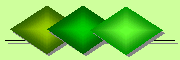Express Accountancy services at affordable prices
 Accounting Property Investment Reporting Company Secretarial Services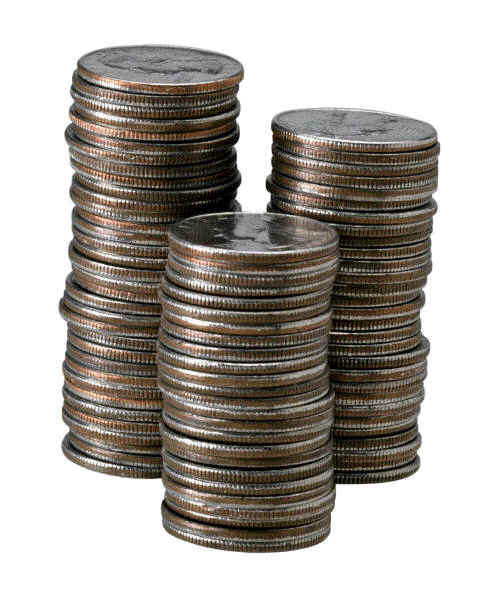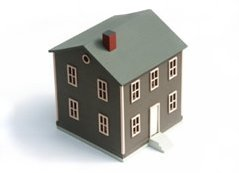Help

Modified Internal Rate of Return (MIRR)

The modified internal rate of return (MIRR) is used to rank property investments of equal size. MIRR is a modification of the internal rate of return (IRR) and addresses the problems with IRR which are:

Reinvestment Decisions
Both the Net Present Value (NPV) and the IRR techniques assume the cash flows generated by an investment property are reinvested within the investment property and at the same rate of return. This is an unrealistic assumption as the funds would probably be reinvested elsewhere if the capital cost of the alternative investment is significantly lower or the return of the alternative investment is significantly higher.

Ambiguity
More than one IRR can be found for investment properties with alternating positive and negative cash flows. MIRR finds only one value as it is the weighted average across investment properties.This can be seen by comparing two hypothetical projects below which generate the same NPV on discounted cash flows and the same MIRR but have different IRR. Both projects have the cost of capital and reinvestement at 10%.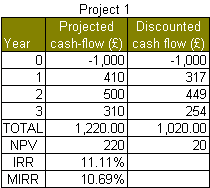For the first project the IRR is 11.11% and for the second project it is 10.69%. However, for both projects the MIRR is the same at 10.69%. The formula for MIRR is:where:
n = power of the root
PV = present value or present discounted value (and not NPV )
i = cost of capital

If we use the figures above then the MIRR formula for either project will contain the following values: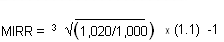where:
power (n) of the root = 3 years
PV = \$1,020
outlay = \$1,000
cost of capital (i) = 10%

The answer for either project is 10.69%. Note that the MIRR here is lower than the IRR value of 11.11 for project 1.

For investment properties , a more realistic scenario is when the investment phase stretches over a number of years. This phase can involve the purchase of land, the erection of a building and any subsquent refurbishments or modifications.

An example is when a plot of land with a derelict building is purchased for \$700,000 in the first year and then is refurbished for \$300,000 in the following year. The expected return on the investment is discounted over the next 3 years. The cost of capital is 10% and the rate of reinvestment is 10%. In order to deal with this scenario we divide it into an investment phase and a return phase.MIRR = 15.66% which is significantly lower than the IRR of 18.81%. As a general rule MIRR values are generally less than IRR figures giving a more conservative estimate of return for investment properties.